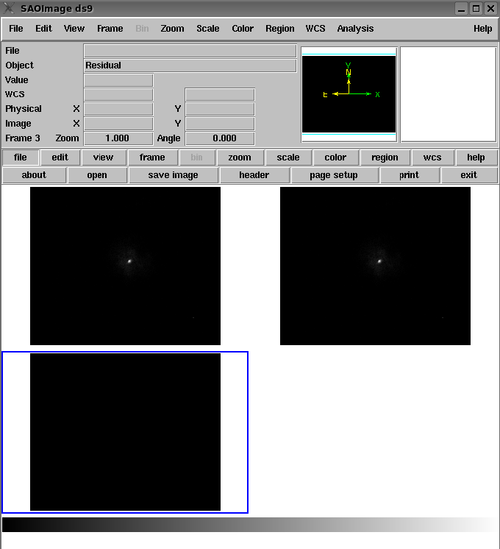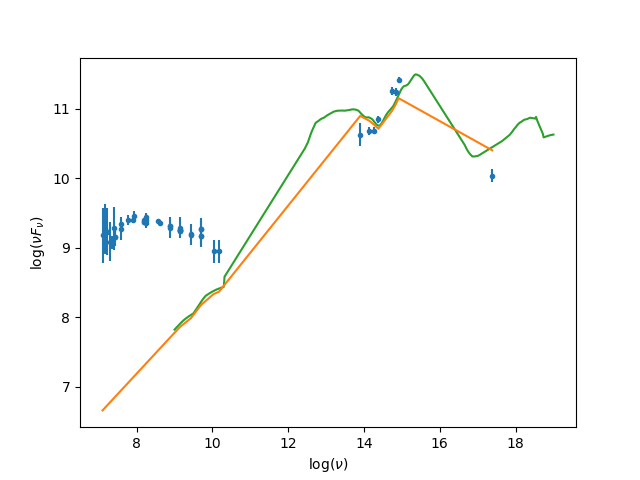# Sherpa Table ModelsSherpa Threads (CIAO 4.11 Sherpa v1)

## Overview

#### Synopsis:

It may be necessary to fit data to a function that is not pre-packaged as a Sherpa model; to do so, we may write that function as a Sherpa "table model". Table models are those that contain data read from a file, and have only one parameter, normalization. When the table model is "calculated", the model returns the data—or more generally, some calculated values based on those data values. "User models" may also be defined in Sherpa; these are models for which the user specifies the parameters and model calculation function. In this thread, examples of table models are shown; for user models, see the thread "Sherpa User Models."

Last Update: 14 Dec 2018 - reviewed for CIAO 4.11, no content change.

## Sherpa Table Models

In addition to the user model class, a table model class has been added to Sherpa; it stores the y-values from an input data file or Crate, and returns them as an array when the table model is asked to "calculate" y-values. The model has one parameter, "ampl", the amplitude; the y-values are multiplied by the amplitude.

In this simple example, we load data from an image, and the resulting Sherpa data set is assigned to a table model:

```
sherpa> print(mytable)
mytable
Param        Type          Value          Min          Max      Units
-----        ----          -----          ---          ---      -----
mytable.ampl thawed            1      -1e+120       1e+120

sherpa> print(mytable.filename)
image.fits

sherpa> print(mytable._TableModel__y)
[ 0.,  1.,  0., ...,  0.,  0.,  0.,]

sherpa> set_model(mytable)
sherpa> image_fit()
```

Here, an instance of the table model is created, and named "mytable". In this simplest example, it is assigned exactly the same data that is read in as the data to be fit. The print function shows that "mytable" has exactly one model parameter, "ampl", which by default is 1.

The model "mytable" also has two attributes, which are not model parameters. The attribute "filename" is the name of the file from which the table data are read, and the attribute "_TableModel__y" simply stores the unfiltered y-values read from that data file. As these are not parameters to be varied during a fit, they are not printed by the call "print(mytable)", which is meant to show a model's parameters; model attributes may be printed with "dir(mytable)".

The y-values which are read from the data file (and stored by the table model class) may be returned as an array when the table model is asked to "calculate" y-values. (Again, since this is not a parameter to be varied during a fit, the array of y-values is not printed by the call print(mytable)).

Then the table model is assigned as a model. The call to image_fit shows that data and model have identical values, and that the residuals are all zero, as expected.

### Figure 1: ds9 image of table model fit to the data contained in 'image.fits'### Figure 1: ds9 image of table model fit to the data contained in 'image.fits'

The Sherpa table model supports linear, nearest-neighbor, and polynomial interpolation of data points on the data set grid from the model grid supplied from file (interpolation is used by the table model to match the data grid to the model grid, which must match before the fit statistics can be calculated for fitting). The grids need not be of constant or comparable bin size, and it is not necessary to match the length of the table model column to that of the data. If the table model grid is not sorted, Sherpa will sort it in ascending order.

### XSpec-style Tables

The load_xstable_model function accepts both additive and multiplicative XSpec-style table models, as demonstrated in the example below.

```sherpa> load_pha("source.pi")
sherpa> print(tab1)
xstablemodel.tab1
Param        Type          Value          Min          Max      Units
-----        ----          -----          ---          ---      -----
xstbl.log mass thawed     0.977121     0.477121      1.47712
xstbl.log lumin thawed           -1           -2            0
xstbl.inc    thawed          0.5            0            1
xstbl.spin   thawed          0.4            0          0.8
xstbl.log alpha thawed           -2           -2           -1
xstbl.norm   thawed
```

In this example, we load PHA data set 'source.pi' and assign to it the multiplicative table model loaded from the file 'bhmodel.fits', which is written in the format required by the XSpec table model (i.e., an N-dimensional grid of model spectra).

## Fitting a Spectrum with a Table Model

In this section, we consider another example of defining a Sherpa table model using model data read from file, but take it a step further and also show the procedure for fitting a 1-D spectrum with the defined model. We consider the Spectral Energy Distribution (SED) template model of radio-loud quasar data in Elvis et al. 1994.

First, we read into Sherpa the data representing the source(s) to be fit, a 3-column × 46-row array of quasar data contained in the ASCII file spectrum.dat. We choose to read in this data array using the Python Crates module, pycrates, and manipulate it within the Sherpa session using Python. (ASCII data can also be read into Sherpa using the Sherpa commands load_ascii/load_table/load_data; see the Sherpa FAQ entry "How do Sherpa 'load' commands handle ASCII files?" for details.)

We assign to variable "dat" the Numpy array which contains our three columns of data fom spectrum.dat: 'Freq', 'Flux' (flux density), and 'Error'. We use Numpy for the mathematical operation of calculating the logarithm of the frequency and flux density columns of the "dat" array, and the Python "array[::-1]" syntax for reversing the order of the arrays. We manipulate the data in this way in order to have it match the SED template model for fitting.

```sherpa> import pycrates as pcr
sherpa> pcr.get_col_names(dat)
['Freq', 'Flux', 'Error']

# convert to log frequency and reverse the order of the array
sherpa> freq = dat.get_column('Freq').values
sherpa> lfreq = np.log10(freq)
sherpa> lfreqr = lfreq[::-1]

# convert to log and reverse
sherpa> flux = dat.get_column('Flux').values
sherpa> lflux = np.log10(flux)
sherpa> lfluxr = lflux[::-1]

# Propagate errors read from the file and reverse
sherpa> err = dat.get_column('Error').values
sherpa> lerr = err/flux
sherpa> lerr2 = lerr[::-1]
```

Here, we have assigned the spectral coordinate array, in frequency (Hz) units, to variable "freq"; the flux density values (Jy-Hz) to "flux"; the errors on the flux density to "err"; and the logarithm of the (reversed) frequency and flux density arrays to variables "lfreqr" and "lfluxr". The "lerr2" array stores the logarithm of the flux density error-to-value ratio. We use these variables as input to the Sherpa load_arrays function in order to assign the loaded data to Sherpa data set ID "1" (the default data set ID).

```sherpa> load_arrays(1,lfreqr,lfluxr,lerr2)

sherpa> show_data()   # equivalent to "show_data(1)"
Data Set: 1
Filter: 7.1004-17.3838 x
name      =
x         = Float64
y         = Float64
staterror = Float64
syserror  = None

sherpa> notice(13,18.)
```

We have also used the Sherpa notice function to restrict the data range to be considered in the fit to the log10(freq) = 13-18 [Hz] range.

Now that the quasar data set has been properly set up for fitting, the next step is to define the model to be used for fitting the data. We use the load_table_model function in the usual way to read in table model data from ASCII file radio_loud.txt, and assign it to model ID "temp". We customize the table model further by freezing its amplitude parameter so that it is not allowed to vary in the fit; this is in order to have the normalization defined as an additive parameter, not multiplicative.

```sherpa> load_table_model("temp","radio_loud.txt")

sherpa> print(temp)
tablemodel.temp
Param        Type          Value          Min          Max      Units
-----        ----          -----          ---          ---      -----
temp.ampl    frozen            1 -3.40282e+38  3.40282e+38

sherpa> freeze(temp)
```

Finally, we use the Sherpa set_model function to define the final model expression for fitting, the sum of the table model "temp" and a constant, the Sherpa const1d model. Since the normalization of the template SED model is frozen, the constant is used to shift this model up and down during the fitting process.

```sherpa> set_model("temp+const1d.c1")
```Using One Source's Spectrum as the Model for Another Source

Suppose there is an interest in using the spectrum of one source (SourceB) as the model fitted to that of another source (SourceA). This can be approached by using a Sherpa table model.

We begin with loading the data and responses for SourceA and SourceB to dataset IDs 1 and 2, respectively, and use the background-subtracted spectrum of SourceB as the model.

```sherpa> load_data(1,"SourceA.fits")

sherpa> subtract(2)
```

In order to define a model compatible with the response files of SourceA, it necessitates deconvolving the corresponding responses from the observed spectrum of SourceB. Numerically, this can be done by dividing the observed spectrum by the detector responses. In Sherpa, the plot_ratio command—which divides the observed spectrum with a model spectrum, the model spectrum being a source spectrum convolved with the ARF and RMF—using a constant source model set to unity, so that the model spectrum is a discrete representation of just the detector responses, may be used for this purpose.

```sherpa> set_source(2,polynom1d.truespec)
sherpa> truespec.c0 = 1
sherpa> photonflux = get_ratio_plot(2).y # ph/s/cm**2/keV
sherpa> energy = get_ratio_plot(2).x # keV
sherpa> photonflux[photonflux < 0] = 0 # replace negative fluxes, if background subtraction is done, with zeroes
```

To cast the energy and photonflux vectors as usable by load_table_model, a table Crate, cr, is defined as the first and second columns, respectively, in the table.

```sherpa> cr = TABLECrate()
sherpa> col1 = CrateData()
sherpa> col1.name = "energy"
sherpa> col1.values = energy
sherpa> col2 = CrateData()
sherpa> col2.name = "photon flux"
sherpa> col2.values = photonflux
```

The unconvolved spectrum of SourceB is now read in as a table model, tt, and then set as the source model for SourceA, convolved with the response files of SourceA.

```sherpa> load_table_model("tt",cr,dstype=Data1D)
sherpa> delete_data(2)
sherpa> set_source(tt)
```

Rather than defining a Crate in memory, writing the table to disk could have also been done easily with save_arrays.

Before fitting the defined model to the quasar data with the Sherpa fit function, we change the minimum value of the constant model to allow for negative "shifts".

```sherpa> c1.c0.min=-100

sherpa> show_model()
Model: 1
(tablemodel.temp + const1d.c1)
Param        Type          Value          Min          Max      Units
-----        ----          -----          ---          ---      -----
temp.ampl    frozen            1 -3.40282e+38  3.40282e+38
c1.c0        thawed            1         -100  3.40282e+38

sherpa> set_stat("leastsq")
sherpa> fit()
Dataset               = 1
Method                = levmar
Statistic             = leastsq
Initial fit statistic = 9960.05
Final fit statistic   = 0.434571 at function evaluation 4
Data points           = 8
Degrees of freedom    = 7
Change in statistic   = 9959.61
c1.c0          -34.2839     +/- 0.353553
```

Finally, we display the full, unfiltered range of the quasar spectrum with the fitted model overplotted, and the errors read in from the data file and defined in variable "lerr2".

```sherpa> notice()

sherpa> plot_fit()

sherpa> set_plot_ylabel("log(freq * Flux_freq)")
sherpa> set_plot_xlabel("log(freq)")
```

### Figure 2: Quasar spectrum fit with binned SED template model### Figure 2: Quasar spectrum fit with binned SED template model

Noting that the plot_fit function shows the model which is binned on the data grid, we decide to overplot the original model using the following set of commands, in order to show the shape better.

```sherpa> templ=pcr.read_file("radio_load.txt[opt skip=2]")
sherpa> templ.get_colnames()
['col1', 'col2']

sherpa> factor0=templ.get_column('col2').values-lfluxr
sherpa> set_curve(["symbol.style","none"])
sherpa> set_curve(["line.color","blue"])
sherpa> set_plot_ylabel("log(freq * Flux_freq)")
sherpa> set_plot_xlabel("log(freq)")
```

### Figure 3: Quasar spectrum with binned and original SED template model### Figure 3: Quasar spectrum with binned and original SED template model

The quasar data spectrum shown with the fitted template model in red (binned on the data grid), and blue (original model).